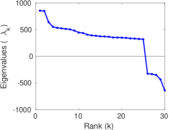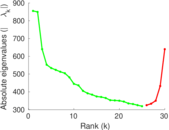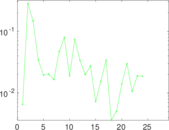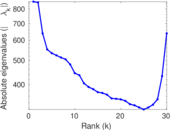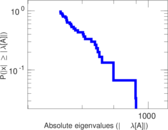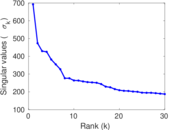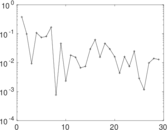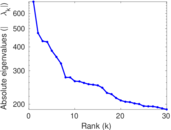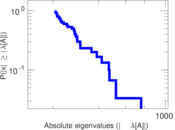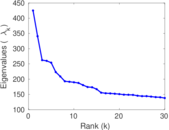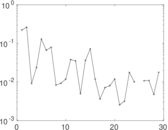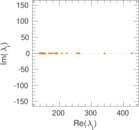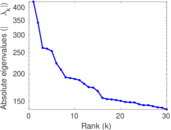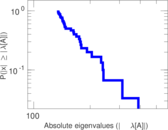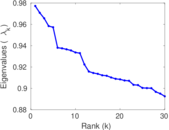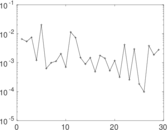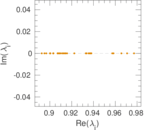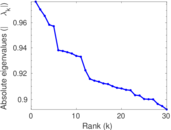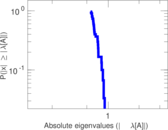This network consists of the wikilinks of the Wikipedia in the Czech language (cs). Nodes are Wikipedia articles, and directed edges are wikilinks, i.e., hyperlinks within one wiki. In the wiki source, these are indicated with [[double brackets]]. Only pages in the article namespace are included.

 Code `Wcs` Internal name `wikipedia_link_cs` Name Wikipedia links (cs) Data source http://dumps.wikimedia.org/ AvailabilityDataset is available for download Consistency checkDataset passed all tests Category Hyperlink network Node meaning Article Edge meaning Wikilink Network format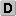Unipartite, directed Edge typeUnweighted, no multiple edges ReciprocalContains reciprocal edges Directed cyclesContains directed cycles Loops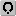Contains loops

## Statistics

 Size n = 655,173 Volume m = 18,516,525 Loop count l = 3,390 Wedge count s = 50,858,040,368 Claw count z = 1,210,571,078,443,897 Cross count x = 3.432 31 × 1019 Triangle count t = 215,915,310 Maximum degree dmax = 153,573 Maximum outdegree d+max = 6,369 Maximum indegree d−max = 153,542 Average degree d = 56.524 1 Fill p = 4.313 68 × 10−5 Size of LCC N = 655,099 Diameter δ = 10 50-Percentile effective diameter δ0.5 = 2.987 09 90-Percentile effective diameter δ0.9 = 4.035 48 Median distance δM = 3 Mean distance δm = 3.498 35 Gini coefficient G = 0.754 233 Balanced inequality ratio P = 0.207 648 Outdegree balanced inequality ratio P+ = 0.238 356 Indegree balanced inequality ratio P− = 0.183 046 Power law exponent γ = 1.420 05 Tail power law exponent with p γ3 = 2.371 00 p-value p = 0.000 00 Outdegree tail power law exponent with p γ3,o = 2.621 00 Outdegree p-value po = 0.000 00 Indegree tail power law exponent with p γ3,i = 2.141 00 Indegree p-value pi = 0.000 00 Degree assortativity ρ = −0.047 162 6 Degree assortativity p-value pρ = 0.000 00 In/outdegree correlation ρ± = +0.781 661 Clustering coefficient c = 0.012 736 4 Directed clustering coefficient c± = 0.211 055 Spectral norm α = 855.217 Operator 2-norm ν = 692.304 Cyclic eigenvalue π = 425.109 Spectral separation |λ1[A] / λ2[A]| = 1.006 56 Reciprocity y = 0.393 006 Non-bipartivity bA = 0.378 374 Normalized non-bipartivity bN = 0.072 688 2 Spectral bipartite frustration bK = 0.000 662 015

## Plots

### Degree distribution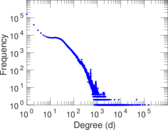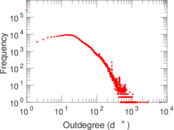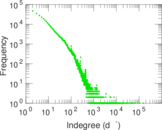### Cumulative degree distribution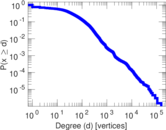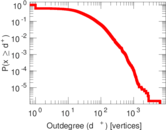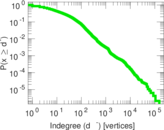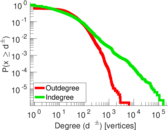### Lorenz curve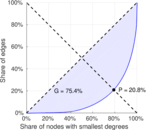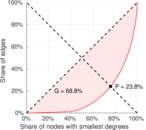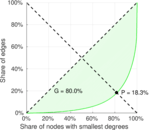### Spectral graph drawing based on the adjacency matrix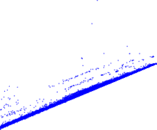### Zipf plot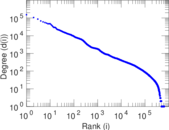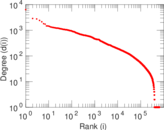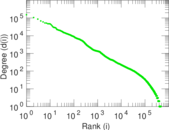### Hop distribution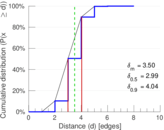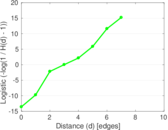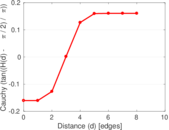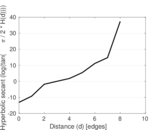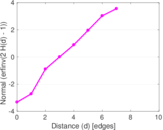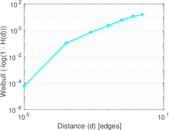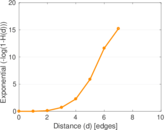### Double Laplacian graph drawing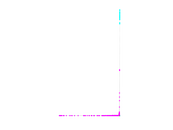### In/outdegree scatter plot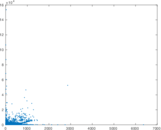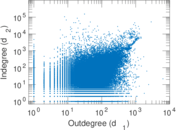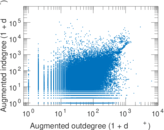### Matrix decompositions plots